{{announcement.body}}
{{announcement.title}}

# Mathematical Functions and Converting Data Types in Groovy

DZone 's Guide to

# Mathematical Functions and Converting Data Types in Groovy

### Learn how to significantly reduce the number of errors allowed in the development of tests that contain mathematical calculations with different types of data.

· Big Data Zone ·
Free Resource

Comment (0)

Save
{{ articles.views | formatCount}} Views

A function is the dependence of one variable on another, or, in simple terms, a function is a definite action on a variable. For example, we take the variable X, perform a certain action with it (for example, exponentiation), and get the variable Y.

In performance testing, mathematical functions are widely used in cases when the logic of the tested object contains mathematical calculations. In this case, testers must perform the mathematical calculations themselves in Apache JMeter™, and then compare them with the result that the tested object returns.

Suppose we have an API that takes two numeric values, multiplies those values, and returns the result to the user. We need to test the correctness of the multiplication operation that the API performs.

When creating the test, two classes will be used:

• Math from the `java.lang` package
• Precision from `org.apache.commons.math3.util`

These classes contain various methods that correspond to mathematical functions and can be used in testing.

In our test, we will create input values for the API in a random way. In addition, numeric values and the result that the API returns can contain a fractional part with three characters (for example, 4,222 x 1,222 = 5,159).

In order to create a test using the methods from these classes, you need to do the following in JMeter.

Right-click > Add > Threads (Users) > Thread Group.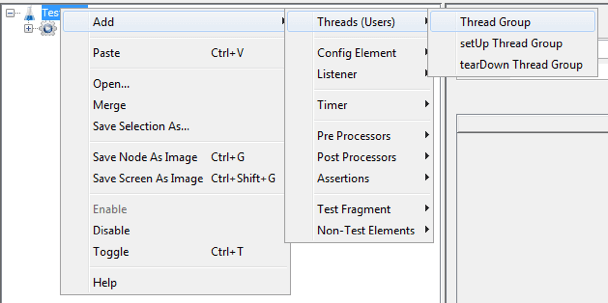## 2. Add a JSR223 Sampler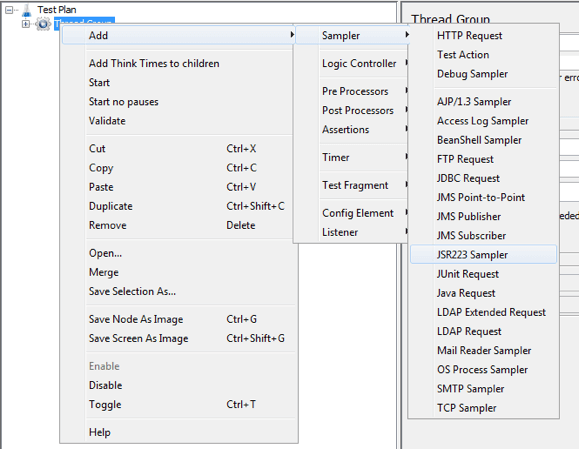JSR223 Sampler > Language Groovy.

In this step, we will create input data for the API and perform a multiplication operation. Then, we will compare our multiplication result to the result that the API returns.

In the JSR223 Sampler, add the following code example.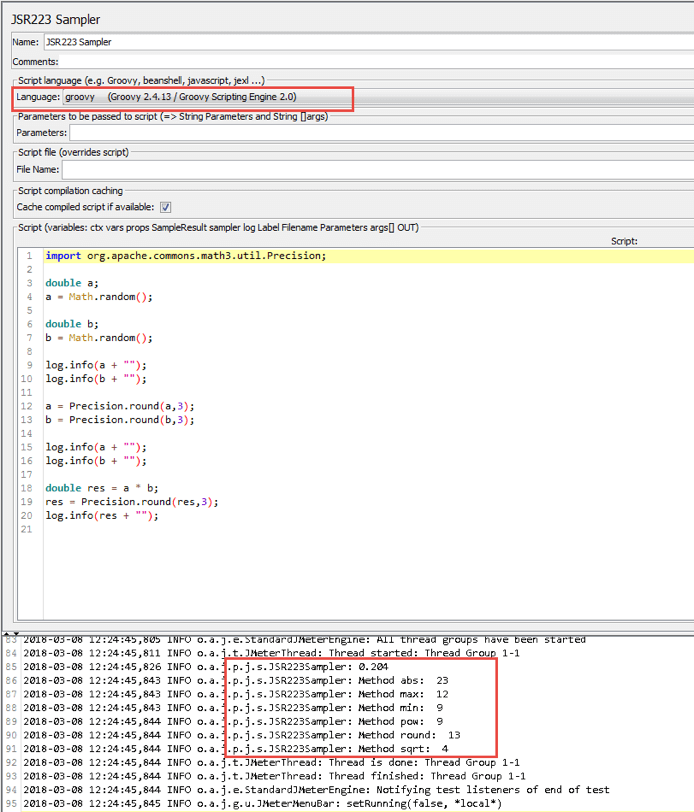This code does the following:

• `import org.apache.commons.math3.util.Precision`: This is the import of class that is necessary for writing code. The class data is imported from the JAR file we added to JMeter.

• `double a` and `double b`: These are variables that are created randomly and will be passed to the API request.

• `a = Math.random(); b = Math.random();`: The method for generating a random number that is greater than or equal to 0 and less than 1. In our case, this method will always generate a number without the integer part (for example, 0.6366686760282807).

• `Precision.round(a,3);`: A method that performs number rounding. The number 3, indicated in the method, means the number of characters after the floating point. In our case, the `Precision.round (a, 3)` method rounds the variable a to 3 characters (e.g 0.324).

• `log.info(res + "");`: A method that outputs data to the console. In our case, this method outputs the value of `res` to the console.

The code shown above can be written more concisely, as shown below: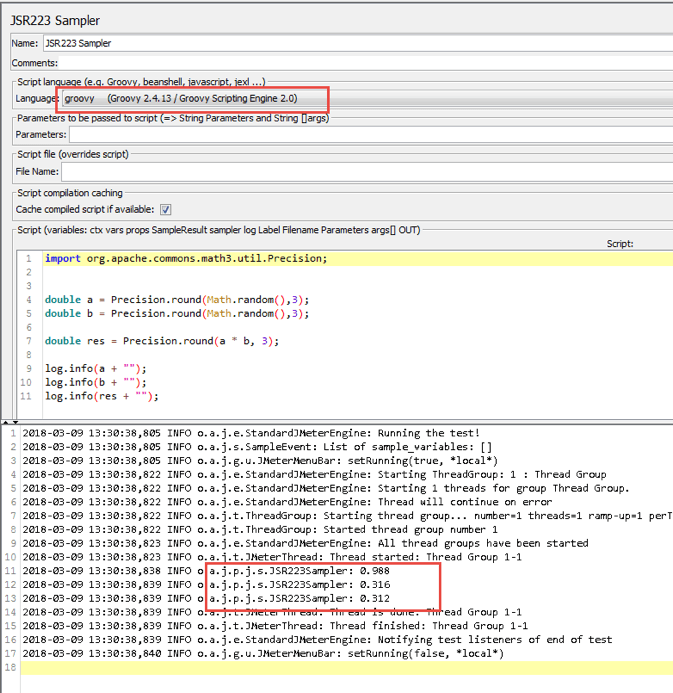Now that the variables are created and their calculation result is executed, it remains for us to pass the variables "a" and "b" to the API request and perform a comparison of our calculation with the result that the API returns.

In the code example above, we created the variables "a" and "b", for which the integer part is "0". In order to create variables with an integer part that is not equal to "0", we need to do the following:

In the JSR223 Sampler, add the following code example.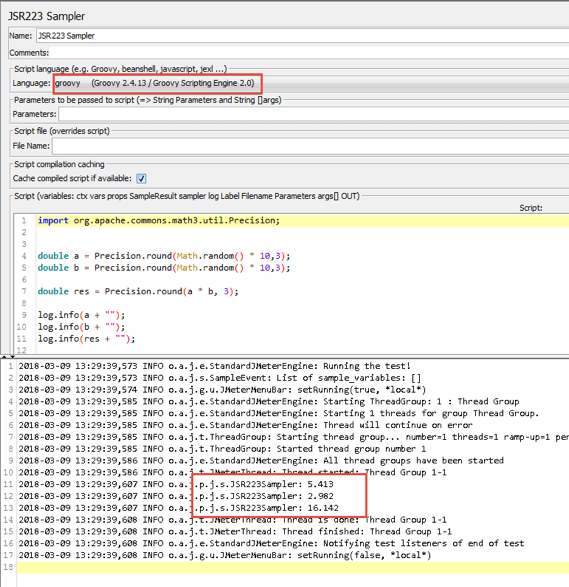This code sample differs from the previous one only in that after the `Math.random ()` method creates a random value, we multiply it by 10. As a result of multiplication, we get variables for which the integer part is not equal to "0".

Below are the methods that are most commonly used when creating automated tests in JMeter:• Math.abs (-23);: A method that returns the absolute value of an argument. An argument is a value that is passed to the method. In our case, the argument is -23.

• Math.max (12, 9);:Returns the maximum argument of two arguments.

• Math.min (12, 9);: Returns the minimum argument of two arguments.

• Math.pow (3, 2): The method of exponentiation. In our case, the number 3 is raised to the power of 2.

• Math.sqrt (16): Extracts the square root of the argument.

The Math class also contains methods for calculating trigonometric and logarithmic functions.

The conversion of numeric data types means changing one type of data to another. A data conversion can be of automatic or explicit type, and it can be performed with loss of accuracy or without loss of accuracy.

## Automatic Data Type Conversion

An example of when you would need automatic conversion is when performing arithmetic operations on variables of different types.

When automatically converting numeric data types, follow these rules:

• If the expression uses variables with the data type byte, short, and char, then these variables are converted to int. The result of the expression will also be of type int.
• If the expression uses a variable with data type long, then all variables are converted to long. The result of the expression will also be of type long.
• If the expression uses a variable with the data type float, then all variables are converted to the float type. The result of the expression will also be of type float.
• If the expression uses a variable with data type double, then all variables are converted to double. The result of the expression will also be of type double.

To perform automatic type conversion, you need to do the following

Thread Group > right-click > Add > Sampler > JSR223 Sampler > Language Groovy.

In the JSR223 Sampler, add the following code example.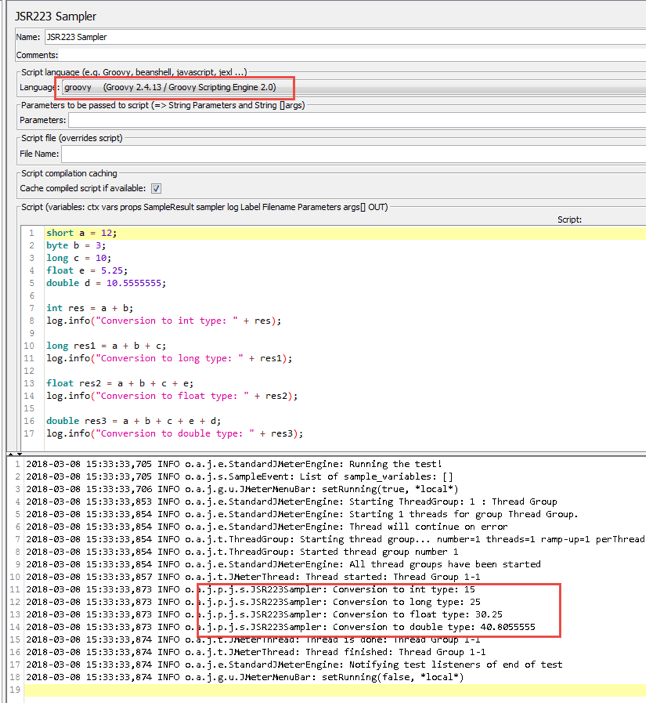## Explicit Data Type Conversion

An explicit conversion occurs when the result of calculation an expression is one data type, and we want to convert it to another type. To perform an explicit conversion of a data type, we must specify the data type before the expression explicitly, for example, (long) (a + b + c). As a result of calculation expression, we get the result with the data type long.

To perform an explicit type conversion, you must do the following:

Thread Group > right-click > Add > Sampler > JSR223 Sampler > Language Groovy.

In the JSR223 Sampler, add the following code example.

## Maintaining and Losing Accuracy When Converting

Automatic and explicit conversion can lead to loss of accuracy, as shown below.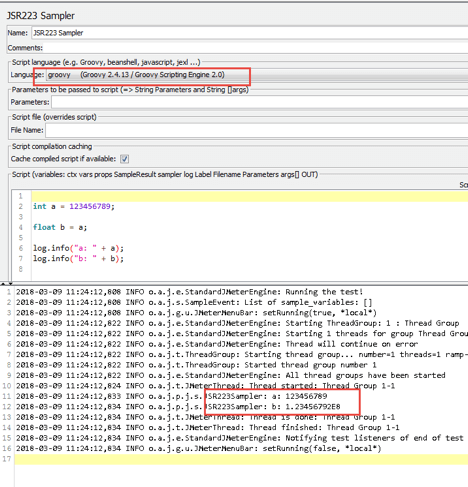The image above shows that after converting the data type int a = 123456789 to float, we get a different number. This is called loss of accuracy.

Variable b has the same order, but with less accuracy.

• a = 123456789
• b = 123456792 (1.23456792E8)

Here is a list of conversions with loss and without loss of accuracy:

• byte to short
• short to int
• char to int
• int to long
• int to double
• float to double

Data type conversions without loss of accuracy:

• int to float
• long to double
• long to float

If we want to convert a data type with a floating point (double) to an integer type (int), then the fractional part will be ignored (the rounding operation will not be performed). An example of such a conversion is shown below.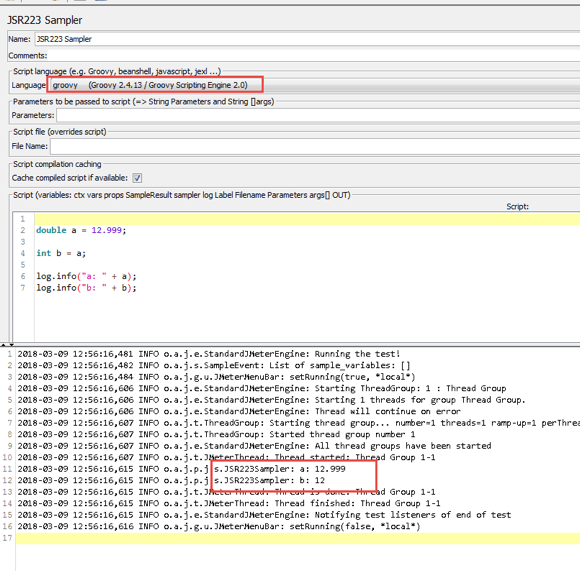In the image above, we see that as a result of conversion double to int, the fractional part was discarded (the rounding operation is not performed).

Given the material in this article, you can significantly reduce the number of errors allowed in the development of tests that contain mathematical calculations with different types of data.

Topics:
big data, data types, groovy, mathematics, thread group, tutorial

Comment (0)

Save
{{ articles.views | formatCount}} Views

Published at DZone with permission of Sergey Horban , DZone MVB. See the original article here.

Opinions expressed by DZone contributors are their own.